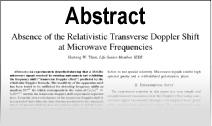Enter the content which will be displayed in sticky bar

# AbstractHigh Velocity Applications of Electrodynamics

Year: 1999
Keywords: Electrodynamics
The problem of the trajectory of a charged particle in both electric and magnetic fields is formulated in three ways: utilizing the Weber equation for the force between moving charges. the classical equation and the new Gaussian equation: In the analysis. in terms of the classic equation, it is necessary to assume that mass varies with velocity. Since both the Weber equation and the new Gaussian equation are expressed in terms of relative velocity, it is possible to retain the simpler concept of constant mass. Applications will be made to the key high velocity configurations. It is not possible to discriminate between the three theories studies on the basis of this set of applications. since the classical theory can be made to conform with real measurements by introducing variation of mass with velocity.